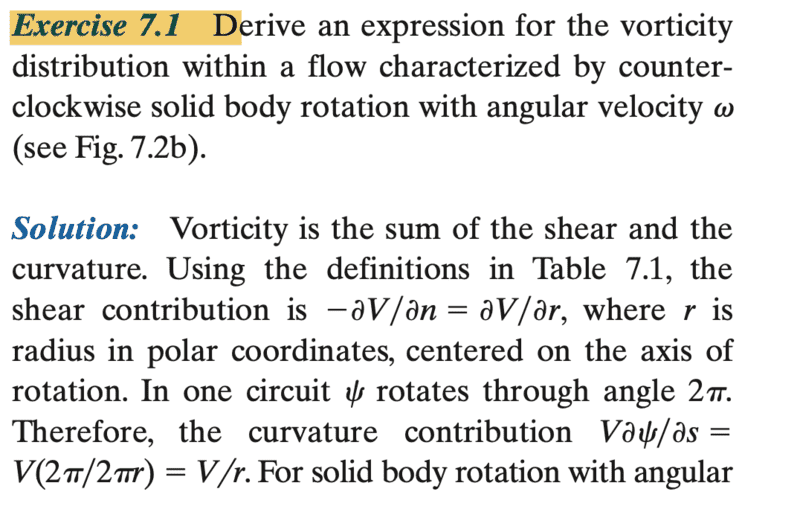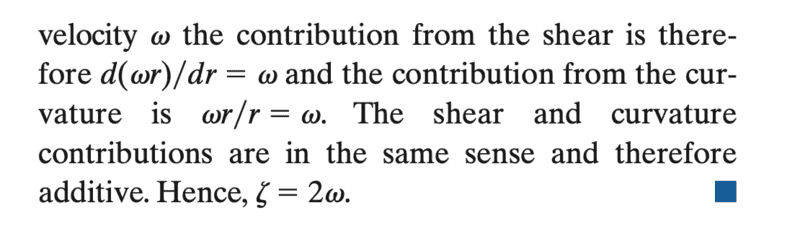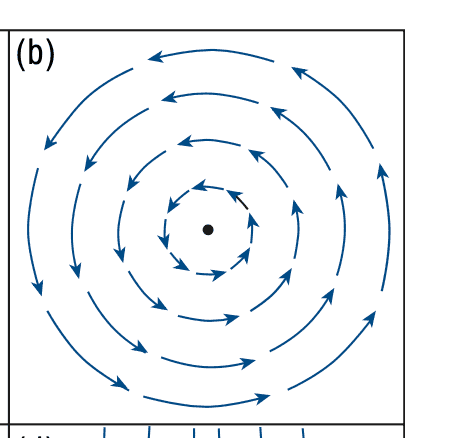# How did they get that the vorticity = 2##\omega##?

Hall
Homework Statement:
Deriving an expression for vorticity.
Relevant Equations:
Vorticity =##2 \omega##
I'm learning Meteorology, and using the book Atmospheric Science by Wallace and Hobbs. We're discussing the kinematics of the winds (fluids). I shall post some images to say what I don't understand. This is how they define their natural coordinate system
At any point on the surface one can define a pair of axes of a sys- tem of natural coordinates (s, n), where s is arc length directed downstream along the local streamline, and n is distance directed normal to the streamline and toward the left,I know and understand the concepts of angular velocity, shear, curvature, differentiation, and partial differentiation but for some reason, which is latent to me, I cannot understand anything that the book has done. Will you please explain it to me?

$$\nabla \times \mathbf{u}=2\mathbf {\omega}$$
$$u_x=- \omega y,\ u_y=\omega x,\,\ u_z=0$$
•